#Interactive Real Analysis

## 7. The Integral

### 7.2. Integration Techniques

This section provides integration techniques, i.e. methods for finding the actual value of an integral. We have already found the most basic technique (the Integral Evaluation Shortcut or First Fundamental Theorem of Calculus): to evaluate an integral over an interval [a, b], find an antiderivate F of the integrand f and compute F(b) - F(a).

 Definition 7.2.1: Antiderivative For a given function f the function F with the property that F '(x) = f(x) is called the antiderivative of f.

Not all functions have a simple antiderivative, and any two antiderivatives of the same function can differ only by a constant (prove this). But different from finding a derivative, where a couple of rules can be used to find virtually every derivative, finding an antiderivative is basically a guessing game.

Example 7.2.2: Standard AntiderivativesFind the following antiderivatives:
 (a)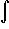xr dx (b)1/x dx (c)ex dx (d)sin(x) dx (e)cos(x) dx (f)tan(x) dx (g)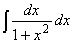(h)(i)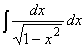As is typical for finding the antiderivatives, the answers for the above examples can not be deduced. They must be guessed, or learned. However, there are two standard mechanisms that can be useful: substitution and integration by parts.
 Theorem 7.2.3: Substitution Rule If f is a continuous function defined on [a, b], and s a continuously differentiable function from [c, d] into [a, b]. Then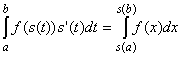This theorem, in words, says that if you can identify a composition of functions as well as the derivative of one of the composed functions, there's a good chance you can find the antiderivative and evaluate the corresponding integral.

Think about the substitution rule as a "change of variable": if a particular expression in x makes an integrand difficult, change it to u. Then compute the derivative of u, i.e. du/dx = u'(x) and "solve" it for du: du = u'(x) dx. If you can use u'(x) dx to change the integrand into an expression involving only u and no x, then the substitution worked (and has hopefully simplified the integrand). Otherwise try a different substitution or a different technique altogether.

 Example 7.2.4: Applying the Substitution RuleHere is a simple example: find(4x + 3)2 dxIf F(x) is an antiderivative of f(x), findf(cx + d) dxx f(cx2) dxf'(x) / f(x) dxCompute the area of a circle with radius r.Findtan(x) dx andcot(x) dxFind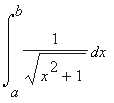and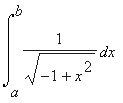Another theorem that is commonly used is "integration by parts".
 Theorem 7.2.5: Integration by Parts Suppose f and g are two continuously differentiable functions. Let G(x) = f(x) g(x). Thenf(x) g'(x) dx = ( G(b) - G(a) ) -f'(x) g(x) dx
In order for integration by parts to be useful, three conditions should be satisfied:
• The integrand must be a product of two expressions (one of which could be 1)
• You must know the antiderivative of one of the two expressions
• The derivative of the other expression should become easier
 Example 7.2.6: Applying Integration by Parts Evaluate the following integrals:x ex dxx2 cos(x) dxln(x) dxsin5(x) dx
Integration by parts can also be used to prove more interesting facts that we might need later.
 Example 7.2.7: Integration by Parts and LimitsSuppose f:[a, b]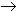R is a continuously differentiable function. Show that: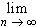f(x) sin(nx) dx = 0f(x) cos(nx) dx = 0
Integration by parts can be used to provide an effective means to compute the value of an integral numerically. Of course, Riemann sums can be used to approximate an integral, but convergence is usually slow. A much faster convergence scheme is based on the trapezoid rule. To prove it, we need the Mean Value Theorem for Integration:
 Theorem 7.2.8: Mean Value Theorem for Integration If f and g are continuous functions defined on [a, b] so that g(x)0, then there exists a number c[a, b] withf(x) g(x) dx = f(c)g(x) dx
Now we can state and prove the Trapezoid Rule.
 Proposition 7.2.9: Trapezoid Rule Let f be a twice continuously differentiable function defined on [a, b] and set K = sup{ |f''(x) |, x[a, b] } If h = (b - a) / n, where n is a positive integer, then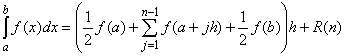where |R(n)| < K/12 (b-a) h2.
The Trapezoid Rule is useful because the error R(n) depends on the square of h. If h is small, h2 is a lot smaller so that the Trapezoid Rule provides a good approximation to the numeric value of an integral (as long as f is twice continuously differentiable).
 Example 7.2.10: Application of the Trapezoid RuleCompare the numeric approximations to the integral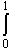sin(x) cos(x) dx obtained by using (a) a left Riemann sum and (b) the Trapezoid Rule, using a partition of size 5 and of size 100.
Our final integration technique uses partial fraction decomposition of a polynomial to simplify rational functions so that they can be integrated.
 Theorem 7.2.11: Partial Fraction Decomposition Suppose p(x) is a polynomial of degree n such that p(x) =, where each qj is polynomial of degree j that is irreducible over R. If s(x) is another polynomial of degree less than n with no factors in common with p(x), then the rational function s(x) / p(x) can be written as a finite sum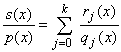where each rj is a polynomial of degree less than j.
This theorem sounds complicated, so we should restate it in a more useful form:
• p(x) is a polynomial that can be factored into polynomials qj
• each qj can not be factored any further (with real coefficients)
• s(x) is another polynomial whose factors, if any, do not cancel any of the qj, and whose degree is less than the degree of p
• Then s(x)/p(x) can be written as a finite sum of simple rational functions whose denominators consist of the qj's and whose numerators have degree at most j-1
• finding the rj amounts to solving a system of linear equations, as the examples will show
Several of the assumptions are technical:
• If the degree of s is greater than or equal to the degree of p, then you can split s(x) / p(x) using long division, and the remainder will be of the form where the theorem applies.
• If s does have factors in common with p, first cancel out those factors, then apply the theorem to the resulting rational function
The next examples will show how partial fraction decomposition can be used to integrate rational functions whose denominator can be factored.
 Example 7.2.12: Integrating Rational Functions To compute the following integrals, use partial fraction decomposition to write the rational function as a sum of simple rational functions.Find the integral(1 - x2)-1 dx where the interval [a, b] does not include 1 and -1.Find the integral(1 - x4)-1 dxFind the integral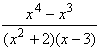dx